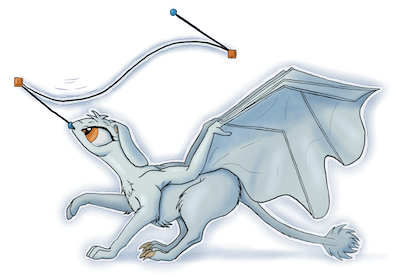## flo_curves

Library for manipulating Bezier curves

### 11 unstable releases

 0.6.1 Mar 6, 2022 Nov 20, 2021 Nov 1, 2021 Apr 29, 2021 Mar 17, 2018

#6 in Rendering

Used in 21 crates (8 directly)

Apache-2.0

1MB
7K SLoC

``````flo_curves = "0.6"
``````

# flo_curves

`flo_curves` is a library of routines for inspecting and manipulating curves, with a focus on cubic Bézier curves. In this library, you'll find routines for computing points on curves, performing collision detection between curves and lines or other curves, all the way up to routines for combining paths made up of multiple curves.

Anyone doing any work with Bézier curves will likely find something in this library that is of use, but its range of functions makes it particularly useful for collision detection or performing path arithmetic.

A set of curve and coordinate types are provided by the library, as well as a set of traits that can be implemented on any types with suitable properties. Implementing these traits makes it possible to add the extra features of this library to any existing code that has its own way of representing coordinates, curves or paths.

`flo_curves` was built as a support library for `flowbetween`, an animation tool I'm working on.

# Examples

Creating a curve:

``````use flo_curves::*;
use flo_curves::bezier;

let curve = bezier::Curve::from_points(Coord2(1.0, 2.0), (Coord2(2.0, 0.0), Coord2(3.0, 5.0)), Coord2(4.0, 2.0));
``````

Finding a point on a curve:

``````use flo_curves::bezier;

let pos = curve.point_at_pos(0.5);
``````

Intersections:

``````use flo_curves::bezier;

for (t1, t2) in bezier::curve_intersects_curve_clip(curve1, curve2) {
let pos = curve1.point_at_pos(t1);
println!("Intersection, curve1 t: {}, curve2 t: {}, position: {}, {}", t1, t2, pos.x(), pos.y());
}
``````

Creating a path:

``````use flo_curves::bezier;
use flo_curves::bezier::path::*;

let rectangle1 = BezierPathBuilder::<SimpleBezierPath>::start(Coord2(1.0, 1.0))
.line_to(Coord2(5.0, 1.0))
.line_to(Coord2(5.0, 5.0))
.line_to(Coord2(1.0, 5.0))
.line_to(Coord2(1.0, 1.0))
.build();
``````

Path arithmetic:

``````use flo_curves::bezier::path::*;

let rectangle_with_hole = path_sub::<_,_, SimpleBezierPath>(&vec![rectangle], &vec![circle])
``````